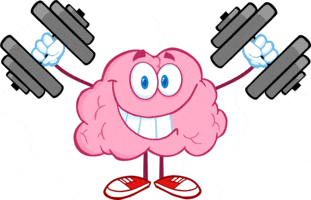# Math Worksheets Land

Math Worksheets For All Ages

# Math Worksheets Land

Math Worksheets For All Ages

Home > Topics >

# Logic Worksheets

This is all about critical thinking. Specifically in logic we are searching for the truth. We display the truth of a statement through the use of true or false. This topic relies heavily on the use of mathematical symbols. We would encourage you to spend a good bit of time working on the use of these symbols with your students. We often find that to lead to more confusion than anything. This basic premise is foundation for many computer programming languages. Logic is, for the most part, void from the core standards, but most schools will include them as precursors for geometric proofs. I feel they are importance enough to recognize. That is why I created this section for you. These worksheets and lessons will help you explore this topic in depth.

### What is Logic in Math?Mathematics is more than just a subject of natural science; it triggers movement in your brain, which wants to understand the nature of the universe. You need strong logic building skills to understand most of the concepts of mathematics. Aristotle suggested different ways to use logic and determine the laws for mathematical reasoning. In other words, the act of contradiction states that the statement can either be true or false and cannot be both of them.

A Venn diagram is a perfect example of logic building and the concept of mathematics. When you are using a Venn diagram, there are two conditions, i.e., "either/or" (inside a single circle only) and "And" (inside both circles). We use either/or when the laws of mathematics provide the options between two or more values. Let us consider an example of x ≤ 5. In this expression, the value of x can be 5 or less than 5. We have options here. Now, if the expression is x = 5. Then, you don't have any choice, and the value needs to be equal to 5 at all costs.

The foundational concept and premise of logic is that it is critically analyzing propositions and statements that are either true or false. In its simplest form, logic is the study or search for truth. Many people believe that it should not be classified as a form of math since it relates directly to many other areas of life. This subject area does give precise meaning to mathematical statements through the use of propositions.

Propositions are the basic unit of logic. They are declarative sentences that can be true or false, but not both. The truth values of them are defined by the uses of the symbols T or F. Obviously standing for true (T) or false (F). Here are some examples of propositions:

1. 4 is a prime number.

2. 845 - 193 = 652

3. A red light means that we must stop.

All of these propositions (above) have a single truth value that indicates is they are valid or invalid. The first proposition is false and the other two are true. If a sentence can not produce a single truth value then it is not classified as a proposition.

As you begin to go deeper with this topic you will come across truth tables. They are charts of columns and rows that display the truth value of every possible combination of a given statement as operated on by logical connectives. Logical connectives are words that are often written as a symbol that directs or operates how a statement is to be followed. The most common forms that we work with are negation, disjunction, conjunction, and implication. Negation is a direct opposition to a proposition. It is often related with the concept (not). Disjunction gives you operational choice of sorts by asserting the concept of (or). Conjunction serves a truth function similar to the word (and) in the English language. An implication is used to establish a relationship between two propositions in which the second is a result of the first proposition.

Unlock all the answers, worksheets, homework, tests and more!
Save Tons of Time! Make My Life Easier Now

## Thanks and Don't Forget To Tell Your Friends!

I would appreciate everyone letting me know if you find any errors. I'm getting a little older these days and my eyes are going. Please contact me, to let me know. I'll fix it ASAP.